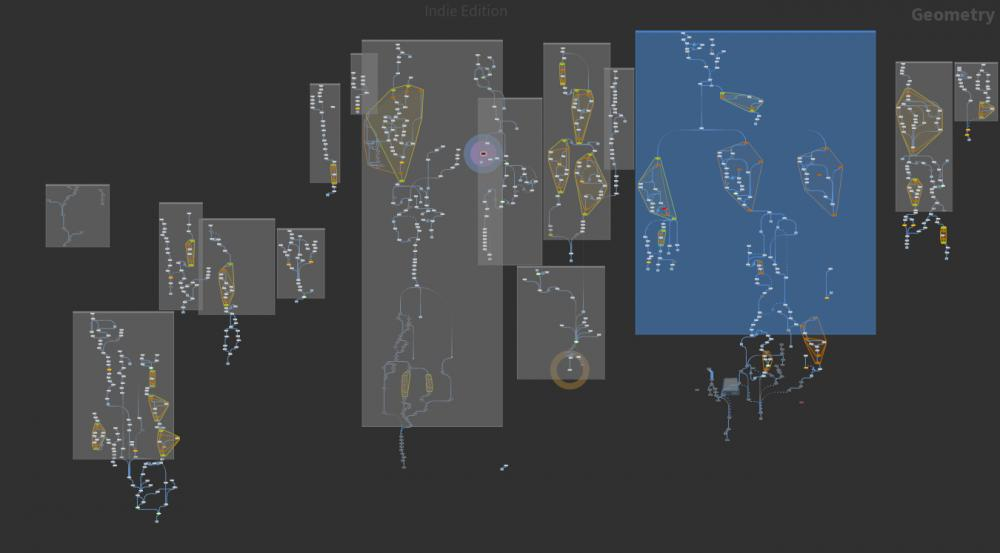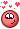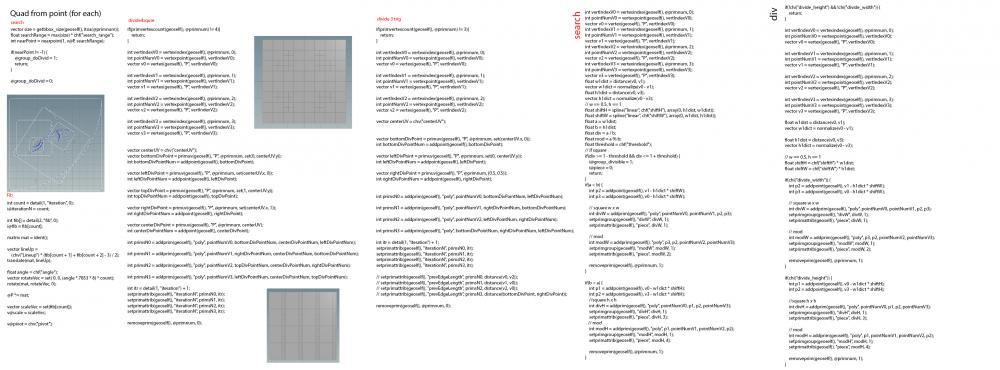[[Template core/front/profile/profileHeader is throwing an error. This theme may be out of date. Run the support tool in the AdminCP to restore the default theme.]]

## Posts posted by Librarian

1. ### Plotter art!!!!

•1
2. ### On Growth and Form

•1
3. ### Learning DOPS, motors, constraints etc

•1

5. ### Sop Solver not working as expected but only after certain frame?

@Sinsterz Please freeze Geometry (include)6. ### [SOLVED] Carve Letter from an Object With Equal Extrusion?

try WRAP SVG Sop from "baku89" on Github

•2
7. ### Tutorial HIP Library

•1
8. ### RBD LookAt Orientation

hm what happens if you make rot attribute and use that in vex like , just wondering..`@orient = eulartoquaternion(radians(@rot),XFORM_XYZ);`

9. ### RBD LookAt Orientation

•2

12. ### attribute access in parameters

@saca attribute rename Mask(or dist) to "height" than you no need to have @ in parameter in Mountain SOP....

if you open(allow editing mountain  Sop you goona see inside VOP why ...you can use falloff node with Blend function also and rest
float blend = point(0, chs("../attrib"), @ptnum);
@P = lerp(@P, point(1, "P", @ptnum), blend);

13. ### array values from parameter

@jon3de You have Bunch of those examples  in 'use' inside package of "Five elements Elf " + many other with arrays ..
mathdeep.vfl
arraydeep.vfl
arithmetic.vfl

•1
14. ### heat, temperature -> which one for blackbody?

```//blackbody
v@temp = blackbody(chf("Temperature"),chf("Intensity"));

//CIE XYZ to sRGB (no white balance)
3@mat3 = { {3.24096994, -1.53738318, -0.49861076},
{-0.96924364, 1.7859675, 0.04155506},
{0.05563008, -0.20397696, 1.05697151} };

//Multiply Matrix
float red = (@temp.x * getcomp(@mat3,0,0)) + (@temp.y * getcomp(@mat3,0,1)) + (@temp.z * getcomp(@mat3,0,2));
float green = (@temp.x * getcomp(@mat3,1,0)) + (@temp.y * getcomp(@mat3,1,1)) + (@temp.z * getcomp(@mat3,1,2));
float blue = (@temp.x * getcomp(@mat3,2,0)) + (@temp.y * getcomp(@mat3,2,1)) + (@temp.z * getcomp(@mat3,2,2));

//set Color
@Cd = set(red,green,blue);```
```//Blackbody
float Temp = chf("Temperature");
float TempInt = chf("Intensity");

float tempX = 0;
float tempY = 0;
float tempZ = 0;

//x
if (Temp >= 1667 && Temp < 4000) {
float xa = -0.2661239 * pow(10,9) / pow(Temp,3);
float xb = -0.2343589 * pow(10,6) / pow(Temp,2);
float xc = 0.8776956 * pow(10,3) / Temp;

tempX = (xa + xb + xc) + 0.179910;

}else if (Temp >= 4000 && Temp <= 25000) {
float xa = -3.0258469 * pow(10,9) / pow(Temp,3);
float xb = 2.1070379 * pow(10,6) / pow(Temp,2);
float xc = 0.2226347 * pow(10,3) / Temp;

tempX = (xa + xb + xc) + 0.240390;
};

//y
if (Temp >= 1667 && Temp < 2222) {
float ya = -1.1063814 * pow(tempX,3);
float yb = -1.3481102 * pow(tempX,2);
float yc = 2.18555823 * tempX;

tempY = (ya + yb + yc) -0.20219683;

}else if (Temp >= 2222 && Temp < 4000) {
float ya = -0.9549476 * pow(tempX,3);
float yb = -1.3481102 * pow(tempX,2);
float yc = 2.09137015 * tempX;

tempY = (ya + yb + yc) -0.16748867;

}else if (Temp >= 4000 && Temp <= 25000) {
float ya = 3.0817580 * pow(tempX,3);
float yb = -5.8733867 * pow(tempX,2);
float yc = 3.75112997 * tempX;

tempY = (ya + yb + yc) -0.37001483;
};

float tempXn = (TempInt / tempY) * tempX;
float tempYn = (TempInt / tempY) * (1 - tempX - tempY);

v@Cd = set(tempXn,TempInt,tempYn);```
```//white point
float wpnX = chf("wpx") / chf("wpy");
float wpnY = chf("wpy") / chf("wpy");
float wpnZ = chf("wpz") / chf("wpy");
vector wpn = set(wpnX,wpnY,wpnZ);

//sort
vector mX = set(chf("priRx"), chf("priGx"), chf("priBx"));
vector mY = set(chf("priRy"), chf("priGy"), chf("priBy"));
vector mZ = set(chf("priRz"), chf("priGz"), chf("priBz"));

//matrix
matrix3 m = set(mX,mY,mZ);
matrix3 mI = invert(m);
matrix3 mW = set(wpnX,wpnX,wpnX, wpnY,wpnY,wpnY, wpnZ,wpnZ, wpnZ);
matrix3 mW2 = mI * mW;

//
vector vecX = set(getcomp(mW2,0,0), 0,0);
vector vecY = set(0, getcomp(mW2,1,1) ,0);
vector vecZ = set(0 ,0 ,getcomp(mW2,2,2));

matrix3 mVec = set(vecX,vecY,vecZ);
3@rgb2xyz = m * mVec;
3@rgb2xyz = invert(@rgb2xyz);

//Color Matrix
float red = (@Cd.r * getcomp(@rgb2xyz,0,0)) + (@Cd.g * getcomp(@rgb2xyz,0,1)) + (@Cd.b * getcomp(@rgb2xyz,0,2));
float green = (@Cd.r * getcomp(@rgb2xyz,1,0)) + (@Cd.g * getcomp(@rgb2xyz,1,1)) + (@Cd.b * getcomp(@rgb2xyz,1,2));
float blue = (@Cd.r * getcomp(@rgb2xyz,2,0)) + (@Cd.g * getcomp(@rgb2xyz,2,1)) + (@Cd.b * getcomp(@rgb2xyz,2,2));

@Cd = set(red,green,blue);```
```//blackbody
v@temp = blackbody(chf("Temperature"),chf("Intensity"));

//set Color
v@Cd = xyztorgb(@temp);```

•1
15. ### [SOLVED]Procedural LetterForm ?

it's huge difference Hobby and commercial,   its your choice...but now from last night  this its the part of OTL's in "Five elements Elf" so you can also have Fun end Share knowledge, instead of just Bubbling.16. ### [SOLVED] Seamless Worley Noise Texture?

@bentraje you forgot in pos-tprocesing to Check Complement.

and you can find on web Nubis noise generator and with Labs you can make endless seamless Noises .

•1
17. ### [SOLVED]Procedural LetterForm ?

@vinyvince I'm just crazy Rabbit .. when I have intersection on any curves and can make polygons with width and having control for these , in combination's I use also golden rules and (quad for each from points) to make endless positions that give me correct " beauty " and space, form and balance between ...here its other Fun Stuff.
Huge Help from Asia and Russian Artist......Indie Zone, GitHub ,Telegram,  and some diff Dark Sites×